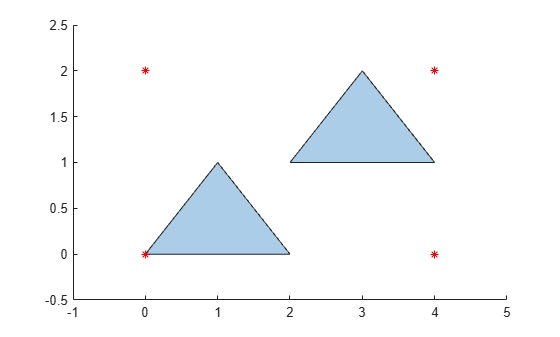# boundingbox

Bounding box of `polyshape`

## Syntax

``[xlim,ylim] = boundingbox(polyin)``
``[xlim,ylim] = boundingbox(polyin,I)``

## Description

example

````[xlim,ylim] = boundingbox(polyin)` returns the x and y bounds of the smallest rectangle enclosing a `polyshape`. `xlim` and `ylim` are two-element row vectors whose first elements correspond to the lower x and y bounds, and whose second elements correspond to the upper x and y bounds.When `polyin` is an array of `polyshape` objects, `xlim` and `ylim` describe the bounding box enclosing all `polyshape` elements of `polyin`.```
````[xlim,ylim] = boundingbox(polyin,I)` returns the bounding box limits of the `I`th boundary of `polyin`.This syntax is only supported when `polyin` is a scalar `polyshape` object.```

## Examples

collapse all

Create a polygon containing two solid regions, and compute the lower and upper x and y bounds that enclose it.

```x1 = [0 1 2]; y1 = [0 1 0]; x2 = [2 3 4]; y2 = [1 2 1]; polyin = polyshape({x1,x2},{y1,y2}); [xlim,ylim] = boundingbox(polyin); plot(polyin) hold on plot(xlim,ylim,'r*',xlim,fliplr(ylim),'r*')```## Input Arguments

collapse all

Input `polyshape`, specified as a scalar, vector, matrix, or multidimensional array.

Data Types: `polyshape`

Boundary index, specified as a scalar integer or vector of integers. Each element of `I` corresponds to a single boundary of the input `polyshape`.

When `I` is a vector of indices, `boundingbox` returns the limits enclosing all boundaries indexed by the elements of `I`.

Data Types: `double` | `single` | `int8` | `int16` | `int32` | `int64` | `uint8` | `uint16` | `uint32` | `uint64`

## Output Arguments

collapse all

x limits bounding a `polyshape`, returned as a two-element row vector. The first element of `xlim` is the lower x bound, and the second element is the upper x bound.

Data Types: `double`

y limits bounding a `polyshape`, returned as a two-element row vector. The first element of `ylim` is the lower y bound, and the second element is the upper y bound.

Data Types: `double`

## Version History

Introduced in R2017b